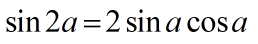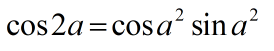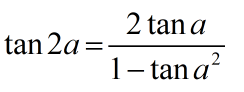Request a Tool

Double Angle Identity Calculator

Double Angle Identity Calculator is use to calculate double angle of sin, cos and tan.

Double angle sin
0
Double angle sin
0

Formula• sin2a = Double angle of sin
• a = Value of angle

Defination / Uses

The doubleangle identities and halfangle identities are special examples of the sum and difference formulae for sine and cosine, respectively. sin2a=2sinacosa

Double angle cos
0
Double angle cos
0

Formula• cos2a = Double angle of sin
• a = Value of angle

Defination / Uses

Cos(2) is always equal to cos2-sin2 according to the cosine double angle formula. For instance, cos(60) equals cos2(30)-sin2 (30). This identity can be used to rewrite phrases or solve difficulties.

Double angle tan
0
Double angle tan
0

Formula• tan2a = Double angle of tan
• a = Value of angle

Defination / Uses

The three common trigonometric ratios: sine (sin), cosine (cos), and tangent (tan) are related with the concept known as a double angle. The term "double" refers to doubling the angle's size.

If you want to get all of the basic double angle identities in one place and calculate them quickly, try our double angle formula calculator. Because you'll need to know and remember these identities to prove, simplify, and solve more sophisticated trigonometric problems, it's critical that you learn and remember them. Don't worry, we'll assist you with this! We've got you covered whether you're looking for the sin double angle formula or the derivation of the cos double angle formula. Keep reading this double angle calculator, and trigonometric identities for double angles will hopefully no longer be a pain in the neck.